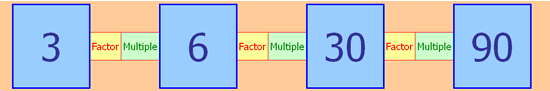Scroll to content

# Week Beginning: November 2nd 2020

Friday 6th November 2020

Investigation Day -

Factor-multiple Chains
Here is an example of a factor-multiple chain of four numbers:Can you see how it works? Perhaps you could make some statements about some of the numbers in the chain using the words "factor" and "multiple".

In these chains, each blue number can range from 2 up to 100 and must be a whole number.
You may like to experiment with this spreadsheet which allows you to enter numbers in each box. Perhaps you can make some more chains for yourself.

What are the smallest blue numbers that will make a complete chain?
What are the largest blue numbers that will make a complete chain?
What numbers cannot appear in any chain?
What is the biggest difference possible between two adjacent blue numbers?
What is the largest and the smallest possible range of a complete chain? (The range is the difference between the largest and smallest values.)

Thursday 5th November 2020

Today we are looking at common multiples. Each factor can make many multiples. Look at the factor 2. It can make 2,4,6,8,10 etc. But what if you look at 2 factors at the same time?

2 = 2, 4, 6, 8, 10, 12, 14, 16, 18

3 = 3, 6, 9, 12, 15, 18

They have what we call common multiples, they both can make some of the same numbers. Complete the sheet below and find as many common multiples as you can.

Wednesday 4th November 2020

Maths Skills -

Complete your weekly maths skills check. Can you get a yellow or green score? Please find the sheet in the document section below.

Tuesday 3rd November 2020

Factors -

https://www.bbc.co.uk/bitesize/topics/zfq7hyc/articles/zp6wfcw

Watch the video above for an explanation of what factors are. These are the numbers that we use to create multiples, for example:

4 x 5 = 20

Here the red numbers are the Factors. The blue number is what we call the multiple, it is a multiple of 4 and 5. However now you can also say that 4 and 5 are factors of 20.

Multiplication uses the commutative law, this means we can switch the factors to solve the problems in other ways.

so 4 x 5 = 20 means 4+4+4+4+4=20.

but 5 x 4 = 20 means 5+5+5+5 = 20

Show what these factors mean as above. Then switch them and show the second meaning:

1. 5 x 6
2. 3 x 7
3. 4 x 2
4. 7 x 8
5. 9 x 4
6. 6 x 10
7. 8 x 8
8. 7 x 6

Top